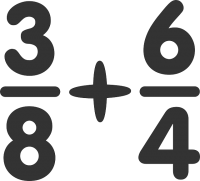# Fractions?

11th October 2017 Off

Fractions is a very important concept in maths.It is defined as part of a whole thing.For example half of a cake or a quarter of a pizza.It is represented in the form of 1/2.In 1/2, 1 is called the numerator while 2 is called denominator.Numerator is used to describe the part of the entity which is in discussion while denominator is used to represent the total number of parts.

## Classifying Fractions.

### Proper Fraction.

A proper fraction has a numerator that is smaller than its denominator, for example 3/7.Proper fraction is always less than 1.e.g 4/5,6/7 etc.

### Improper Fraction.

An improper fraction has a numerator that is bigger than its denominator, for example 10/7.Improper fraction is always greater than 1. e.g 16/7,13/9 etc.If numerator is equal to denominator then the fraction is equal to 1.These  are also called improper fractions e.g. 5/5=1,4/4=1 etc.

### Mixed Fraction.

A fraction which is  represented as 2  1/4 is called a mixed fraction because it has a whole number and a fraction together.

## Comparing Fractions

### Common fractions.

A fraction in which numerator and denominator both are integers is called common fraction.e.g. 5/7 is a common fraction while 1/2/3/5 is not common fraction here numerator  and denominator are also a fraction i.e 1/2 and 3/5.

### Equivalent Fractions.

Some fractions may look different, but are really the same,so these are called equivalent fractions. for example:

 4/8 = 2/4 = 1/2 (Four-Eighths) (Two-Quarters) (One-Half)

It is always desirable to show an answer in its simplest possible way. In this above examples it is 1/2.This process is called simplifying or reducing a fraction.

#### 1 Least Common Denominators(LCD).

In Maths, the lowest common denominator or least common denominator (abbreviated LCD) is the least common multiple of the denominators of a set of fractions.LCD of a set of fraction is the lowest number which we can use in the denominators to convert these fractions into equivalent fractions that have the same denominators.

e.g

1/3 and 4/5 are two fractions.In order to create the same denominator of both fractions,we  convert them into their equivalent fractions in such a way that both of them have a common denominator.We find the LCD of fractions to find equivalent fractions. In the above example,  5/15 and 12/15  are equivalent of 1/3 and 4/5. if we find their Least Common  Multiplication (LCM) which became their LCD and it is used to create the Equivalent Fractions.

#### 2.Least Common Multiplication (LCM).

A common multiple is a number that is a multiple of two or more numbers. The common multiples of 3 and 4 are 0, 12, 24, .... The least common multiple (LCM) of two numbers is the smallest number (not zero) that is a multiple of both.So the LCM of 3 and 4 is 12.

## Basic Math Operations.

1-With Common Denominator:We add the numerators and then simply it at the end  to get the addition e.g

A)2/7+4/7+5?7=11/7.

B) 5/8+1/8=6/8=3/4. (divide by 2 to simplify it.)

Addition of fractions with common denominators can be practiced here using our worksheets for year 4.

All our worksheets are designed on the basis of  latest national curriculum.

2-With different Denominators:If denominators of the fractions are different then we use the following method.

e.g. 3/8+1/4=??

Method:We must somehow make the denominators the same.

In this case it is easy, because we know that 1/4 is the same as 2/8 :

 3/8 + 2/8 = 5/8

If we cannot find the suitable equivalent fraction we have to use  following  method to find the common denominators and then add them later.

#### How to Find Addition of fractions  by LCD method?

1. First,convert all the integer and mixed numbers into proper fractions.
2. Then for all the denominators, find the LCM(Least Common Multiplication).
3. This LCM number will be LCD  of  set of fractions.
4. Now divide the LCD found in step 3 by each of the denominators.
5. After that multiply both the numerator and denominator by the number found in step 4.
6. In the end,add the numerators of the newly found fractions from step three but keep the same denominators.
7. This will give the result of addition of  set of fractions.

Example.

4/5+ 6/7=?

• First find the LCM of both denominators which  is 35.
• Now Use this LCM to make LCD and divide 35 by 5 and  get 7.Then divide 35 by 7 and get 5.
• After that multiply both numerator and denominator separately by their respective number. In this case,multiply  7 by 4 and then by 5  e.g. 4*7/5*7=28/35.Similarly 6*5/7*5=30/35.
• Add the new numerators of both fractions by keeping the denominators same to get the result. i.e  28/35+30/35=58/35.answer.

### Subtraction.

The method of subtracting the fractions is same like addition.If denominators  are same then subtract the numerators like simple subtraction e.g. 5/4-3/4=2/4=1/2.

If denominators are not equal then first make them equivalent fraction using LCD method of equivalent fractions.

### Multiplication .

Division.
This is a very useful description of fractions in a BBC website.